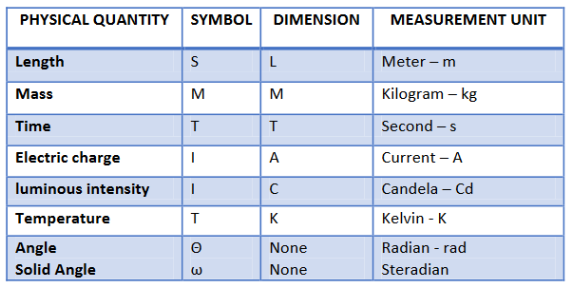# Units & Measurements

If Q is the quantity, n is the numerical value and u is the unit then;

Q = nu

n1u1 = n2u2 (bigger the unit, smaller is the value)

Systems of Measurement

1) CGS (Centimetre-Gram-Second) system.

2) FPS (Foot-Pound-Second) system.

3) MKS (Meter-Kilogram--Second) system

4) MKSA (Meter-Kilogram-Second-Ampere) unit.

Fundamental Units

These units are independent of other units, thus called as fundamental units.Dimensional Formula:-

Dimensional formula of a physical quantity is the formula which tells us how and which of the fundamental units have been used for the measurement of that quantity.

How to write dimensions of physical quantities: -

1) Write the formula for that quantity, with the quantity on L.H.S. of the equation.

2) Convert all the quantities on R.H.S. into the fundamental quantities mass, length and time.

3) Substitute M, L and T for mass, length and time respectively.

4) Collect terms of M, L and T and find their resultant powers (a, b, c) which give the dimensions of the quantity in mass, length and time respectively.

Uses of Dimensional Analysis:

1) To check the correctness of an equation

2) To find the conversion factor

3) To find the dimensions by comparison method

Significant Figures

There are three rules on determining how many significant figures are in a number:

1) Non-zero digits are always significant.

2) Any zeros between two significant digits are significant.

3) A final zero or trailing zeros in the decimal portion ONLY are significant.

4) If the last digit to be rounded off is 5 or greater than 5, then the last significant digit is increased by 1.

Order of Magnitude

Order of Magnitude is the power to which 10 is raised to; to indicate the size of the quantity .

To find out order of magnitude:

1) The number should be in unit’s place. e.g. 2.15 x 104has the order 4.

2) If the number in unit’s place is 5 or greater than 5, then increase the order by 1. e.g. 7.4 x 106has the order 7.

Types of Errors

Personal Error: Error occurring due to human errors.

Random Error: Error occurring due to random changes in the environment of the experiment.

Systematic Error: Error occurring due to a constant error in the readings of instruments. This is caused due to the faulty calibration of instrument.

Instrumental Error: Error occurring due to faulty construction of instrument.

Error Analysis

Mean Value: Average of All readings

Absolute Error: Individual Reading -Mean Value

Mean Absolute Error: Average of all Absolute values

Relative Error: Mean Absolute Error / Mean Value

Percentage Error: Relative Error x 100

Rules for Error Analysis:

1) If dx is the error in x, then Error in xn is n dx.

2) If dx is the error in x and dy is the error in y, then the Error in x+y, x–y, xy, x/y, is dx+dy

Post By : Ravindra Yadav 02 Nov, 2019 3323 views Physics